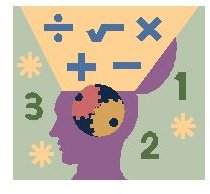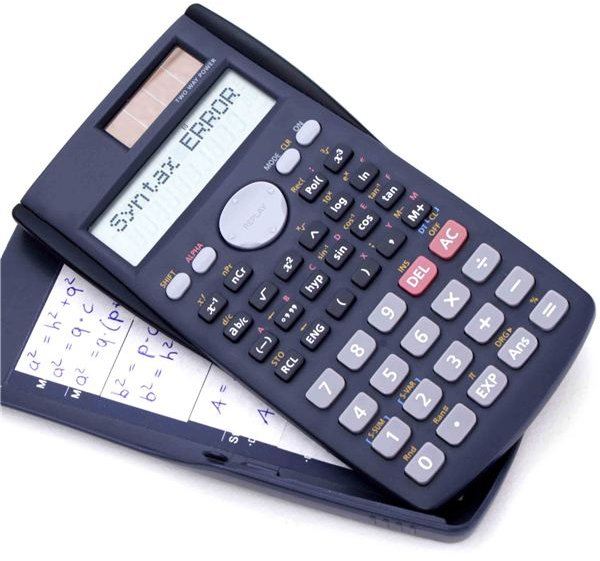# 4th Grade Math Word Problems: Strategies, Ideas and Examples for Teachers

## Characteristics

Solving word problems takes skill, attention to detail, and a good problem solving strategy. Fourth grade math word problems usuallyinvolve one of the basic math operations – addition, subtraction, multiplication, or division. It is not uncommon to see two operation types in one problem, but generally speaking there is often only one operation involved. The word problems themselves require either a one or two step calculation to correctly solve the question, and they are characterized by real world scenarios familiar to a 4th grade student.

## ExamplesA one-step problem may be as simples as, "Jack has \$4.25 and Kayla has \$3.80. How much money do they have altogether?" This straightforward problem merely requires the students to add the two amounts of money together. At the beginning of fourth grade, this would be an appropriate example of 4th grade math word problems. A more advanced one-step problem would be, "A friend tells you that they will be 405 weeks old on their next birthday. How old is that in years?"

Two-step word problems require more effort. For instance, "Michael ate 12 cookies, while his sister ate 9 cookies. If mom baked 56 cookies, how many are left?" Again this would be a word problem students may encounter at the beginning of 4th grade. More taxing problems could include the likes of, "A sign before a bridge says 'weight limit 2 Tons.' The pickup truck weighs 1,675 lb, and has a bag of sand that weighs 400 lb in the back. The driver weighs 175 lb. Should he drive the truck across the bridge?"

## Strategies

There are many different strategies for solving this type of problem, but the one that I have had the most success with is a four-step problem solving strategy.

1. Understand: What are you being asked to find out? Students need to identify the outcome of the problem and keep this in mind as they are working towards a solution.
2. Plan: What are you going to have to do to solve this problem? Will you need to guess and check? What operation(s) are involved? Will you draw a picture to help you? Can you make an estimate?
3. Solve: If the plan is sound, then this is the stage where you put it into action